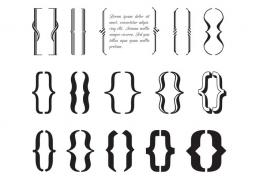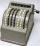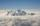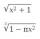# Brackets 2

5-2×6-4+2=5

Result

x = (Correct answer is: 5-2×(6-(4+2)))#### Solution:

$x=5-2×\left(6-\left(4+2\right)\right)$We would be pleased if you find an error in the word problem, spelling mistakes, or inaccuracies and send it to us. Thank you!## Next similar math problems:

• NoontimeIn the morning temperature was 110°F. By noontime it has gone up by 15°F. What was the noon temperature. (Give your answer in °F)Combine like terms 4c+c-7c
• OperationsSum of the numbers 1.01 and 3.35 multiply by the difference of numbers 6.69 and 1.39.
• BarrelsPeter has 42 barrels. One-sixth of them are filled with lemonade, one third is filled with wine and half is empty. Calculate how many barrels are with each filling.
• Algebra problemThis is algebra. Let n represent an unknown number. 1. Eight more than the number n 2. Three times the number n 3. The product of the number n and eight 4. Three less than the number n 5. Three decreased by the number n
• Expression 1What is 7+8-(5×2)+5-4+(6×(5-3)+6)-(8+10)-7+6?
• The temperature 3The temperature is -12 degrees Fahrenheit. During the following 5 hours the temperature decrease 23 degrees Fahrenheit what is the temperature at 11 pm?
• Evaluate expression 2Evaluate expression with negatives: (-3)+4+(-8)+(-6)+4+(-1)
• CupcakesIn a bowl was some cupcakes. Janka ate one third and Danka ate one quarter of cupcakes. a) How many of cookies ate together? b) How many cookies remain in a bowl? Write the results as a decimal number and in notepad also as a fraction.
• MountainMountain has the elevation 7450 meters and in the morning is the middle portion thereof in the clouds. How many meters of height is in the sky if bellow the clouds is 2,000 meters and above clouds are two fifths of elevation of the mountain?
• EricaErica bought 3 1/2 yards of fabric. If she uses 2/3 of the fabric, how much will she have left?
• Product of the sum and differenceCalculate the product of the sum and difference of numbers -7 and -2.
• OpheliaOphelia recorded the temperature of a cold store every two hours. 1. At 6am it was -4°C and at 8am it was -1°C. By how much did the temperature rise? 2. The temperature went up by 5°C in the next two hours. .What was the temperature at 10am?
• Evaluate expressionIf x=2, y=-5 and z=3 what is the value of x-2y
• With bracketCalculate (evaluate) simple mathematical expression with a negative numbers and a bracket: 13+15*5-2*(-6)
• Temperature rise and fallOn Friday, the temperature was 82°F. The temperature changed by –2°F on Saturday, and then it changed by 5°F on Sunday. What was the temperature on Sunday? How did the temperature change? Note we consider the mathematical problem formulated in this way to
• LaboratoryMang Elio went to his laboratory at 7:00am And recorded that the temperature was 11.07°C. At lunchtime, the temperature rose by 27.9°C but dropped by 8.05°C at 5:00 PM. What was the temperature at 5:00 PM?